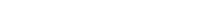# Math riddles Level 8 Answer with Hints and solutionsMath riddles game level 8 Answer with solution android game developed by Black games. Scroll Below to find details.

Math Riddles tests your IQ with mathematical puzzles. Challenge yourself with different levels of math puzzles and stretch the limits of your intelligence. Every IQ game is prepared with an approach of an IQ test. Improve your mathematics, challenge your brain with this game. There are many hard levels which you may not have solved then you can take help from here.

Math app level 8: A+B=60

A-B=40

A/B=?Solution: A=50

B=10

then A/B=50/10=5

If there is any Doubt/discrepancy then please let me know in comments or you are unable to understand solution then I will surely help you.

### 8 thoughts on “Math Riddles App Level 8 Solution Android”

•August 26, 2019 at 11:34 pm

Doesn’t 2 also work?

40 + 20 = 60
60 – 40 = 20
40/20 = 2

•August 26, 2019 at 11:35 pm

Scratch that. Just noticed my error!

•July 11, 2019 at 12:50 am

•June 13, 2019 at 5:19 pm

I want to make many questions just like this. So could you pliz write the algorithm of only this problem

•June 14, 2019 at 12:14 am

Best idea to Take any two numbers such taht 25 and 5
So question becomes
A+B=30
A-B=20
then A/B=?

•March 9, 2019 at 12:08 am

No tiene sentido. Sí A+B es 60 y A-B es 40 quiere decir que B es igual a la diferencia entre esos dos resultados. Por ende A=40 y B=20. Es decir que A/B= 4

•January 27, 2019 at 6:41 pm
•